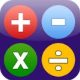# Math Is Fun Forum

Discussion about math, puzzles, games and fun.   Useful symbols: ÷ × ½ √ ∞ ≠ ≤ ≥ ≈ ⇒ ± ∈ Δ θ ∴ ∑ ∫  π  -¹ ² ³ °

You are not logged in.

## #1 2013-05-31 19:16:26

Hostible
MemberRegistered: 2013-05-31
Posts: 5

### Supplement Multiplication

SUPPLEMENT MULTIPLICATION
[The supplement of a number is the amount by which that number is more than 100]

Find the product of 104 x 106.

(a) Add one of the numbers to the supplement of the other number.
104 + 6 = 110 or 106 + 4 = 110
These are the first two digits of the final product.

(b) Multiply the supplements of the two numbers together. 4 x 6 = 24.
These are the last two digits of the final product.

106 x 104 = 11024

Offline

## #2 2014-12-12 00:20:57

DeanPemberton
Member
From: USA
Registered: 2014-10-30
Posts: 20
Website

### Re: Supplement Multiplication

Wow, it is a great formula, maths always rocks. You don't need any calculator or long calculation for multiplying two numbers which is more than 100.

Offline